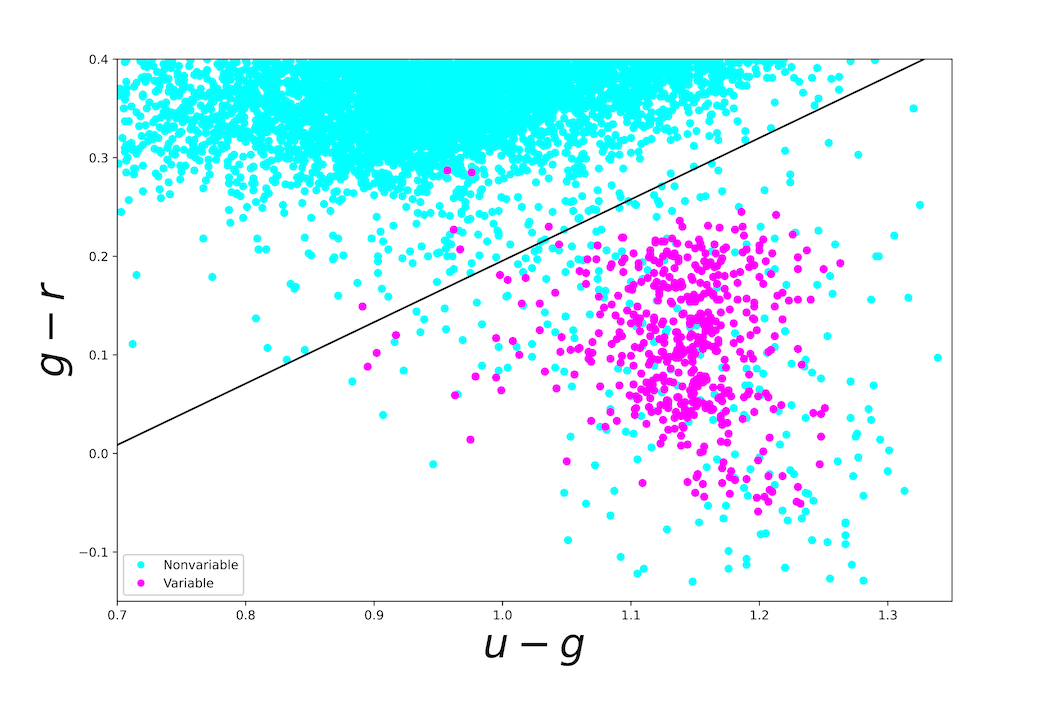# Analyzing Astronomical Data with Machine Learning Techniques

Status Report
astro-ph.IM
February 24, 2023Logistic Regression classification method is used to separate variable stars from nonvariable stars. The light blue points show nonvariable sources, while the violet show variable sources. The classification boundary is shown by the black line. Logistic Regression attains an accuracy of 0.969 and a F1-score of 0.628.

Classification is a popular task in the field of Machine Learning (ML) and Artificial Intelligence (AI), and it happens when outputs are categorical variables. There are a wide variety of models that attempts to draw some conclusions from observed values, so classification algorithms predict categorical class labels and use them in classifying new data.

Classification is a popular task in the field of Machine Learning (ML) and Artificial Intelligence (AI), and it happens when outputs are categorical variables. There are a wide variety of models that attempts to draw some conclusions from observed values, so classification algorithms predict categorical class labels and use them in classifying new data.

Popular classification models including logistic regression, decision tree, random forest, Support Vector Machine (SVM), multilayer perceptron, Naive Bayes, and neural networks have proven to be efficient and accurate applied to many industrial and scientific problems.

Particularly, the application of ML to astronomy has shown to be very useful for classification, clustering, and data cleaning. It is because after learning computers, these tasks can be done automatically by them in a more precise and more rapid way than human operators.

In view of this, in this paper, we will review some of these popular classification algorithms, and then we apply some of them to the observational data of nonvariable and the RR Lyrae variable stars that come from the SDSS survey. For the sake of comparison, we calculate the accuracy and F1-score of the applied models.

Comments: Proceedings based on the lectures given at the hands-on workshop of the ICRANet-ISFAHAN Astronomy Meeting, to be published in Astronomical and Astrophysical Transactions
Subjects: Instrumentation and Methods for Astrophysics (astro-ph.IM); Solar and Stellar Astrophysics (astro-ph.SR); Computational Physics (physics.comp-ph); Data Analysis, Statistics and Probability (physics.data-an)
Cite as: arXiv:2302.11573 [astro-ph.IM] (or arXiv:2302.11573v1 [astro-ph.IM] for this version)
https://doi.org/10.48550/arXiv.2302.11573